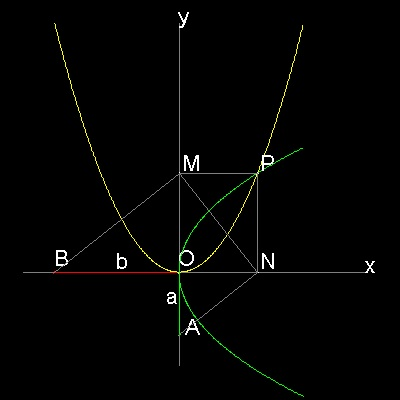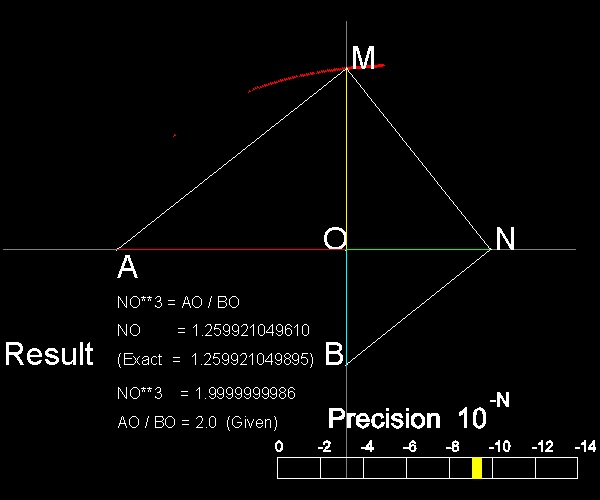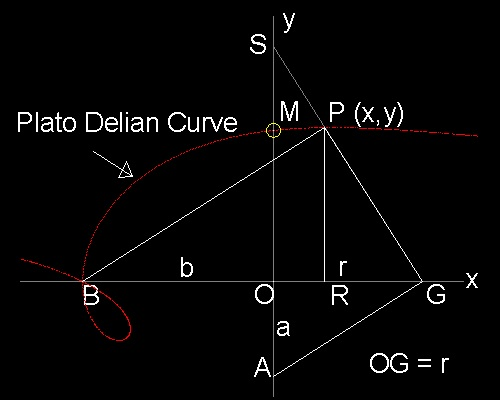Doubling the Cube
Go to   Fun_Math Content Table   Three Famous Problems   Delian Problem

### Doubling the cube by Greek Mathematicians

#### Plato's Cube doubling Tool

The basic concept of Menaechmus's method is to rotate three similar right triangles around one point "O" clockwise starting from BO.(See the #3 case of Menaechmus below).There are multiple ways to accomplish this motion by mechanical constructions.

One way is as follows:
ANM is a rigid bar with angle ANM set to 90 degrees.
Bar BM moves in such a way that it always stays perpendicular to MN.
Restrictions: BM passes through point B, and AN passes through point A.

********** Menaechmus_Delian_3.dwg *********

A sample is shown in the next figure.********** Plato_Delian_tool.dwg *********

A (Japanese) carpenter's square is used as a rigid 90 degrees tool, and a drafting triangle is used to slide along this square.
For reference, exact solutions are
ON = (2)1/3 = 1.2599.. , and OM = (2)2/3 = 1.5874..
In the figure, ON =1.25, and OM = 1.55 ~ 1.6

#### Plato's Delian Solution********** Plato_Delian_result.dwg *********

You can see the process in animation

To create this drawing and animation:
Then from command line, type Plato_Delian

#### Curve of the point M

The graphical soution above also plots point "M" in red color. The process continues until the point comes closer within the pre-specified criteria of convergence(1.e - 9).
Let us find out what kind of curve these points lie on.********** Plato_Delian_curve.dwg *********

 ``` BPGA is the Plato's apparatus. G is on x-axis, but P is not on y-axis. PR is drawn perpendicular to x-axis, and extend GP to meet y-axis at S. In the drawing, AO = a, BO = b, PR = y, OR = x, and OG = r In the right trianlge BGP, BR.RG = PR2 or (b+x)(r-x) = y2 (1) Since trianlgle SOG and PRG are similar, PR:RG = SO:OG = OG:OA or y/(r-x) = r /a (2) Eliminating r from (1) & (2), we obtain,as the locus of point P (x,y), the following equation of curve. a(b+x)2 = y(x2 + y2 + bx) (3) This is a curve of cubic terms in y, and shown in red dot in the drawing. A very interesting shape ! The solution point (M) is the intersection of this curve and y-axis. It is given by letting x = 0. Therefore y3 = OM3 = ab2 Note here that if we choose line BPG as a rigid frame and try to find point G ,then the equation will be obtained by changing a to b, x to y in (3) . b(a+y)2 = x(x2 + y2 + ay) The intersection is obtained by letting y = 0. or ba2 = x3 = ON3 ```

To create this drawing :